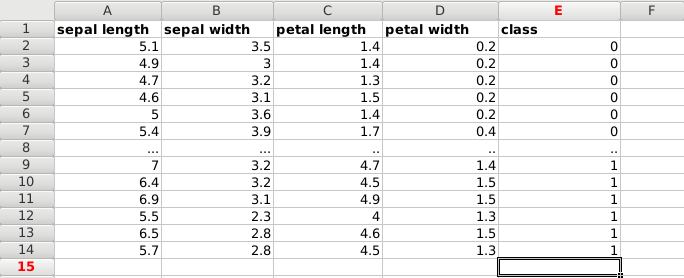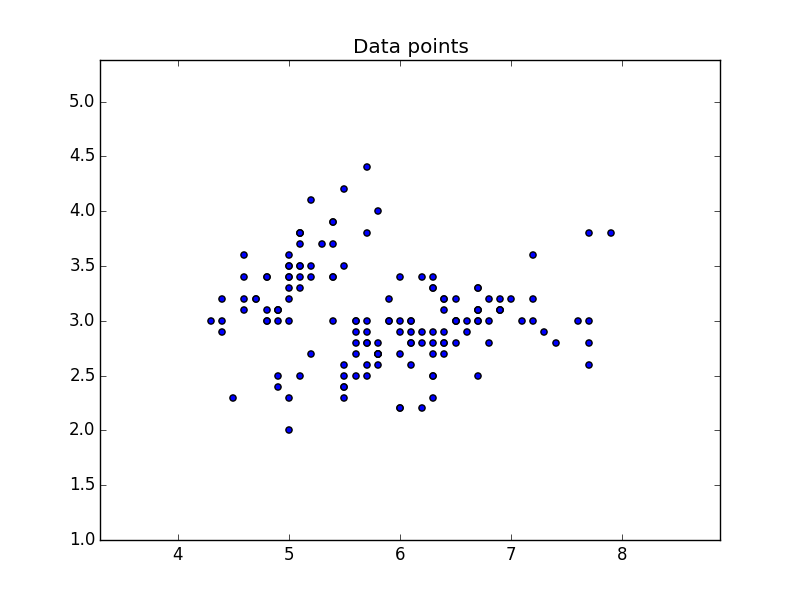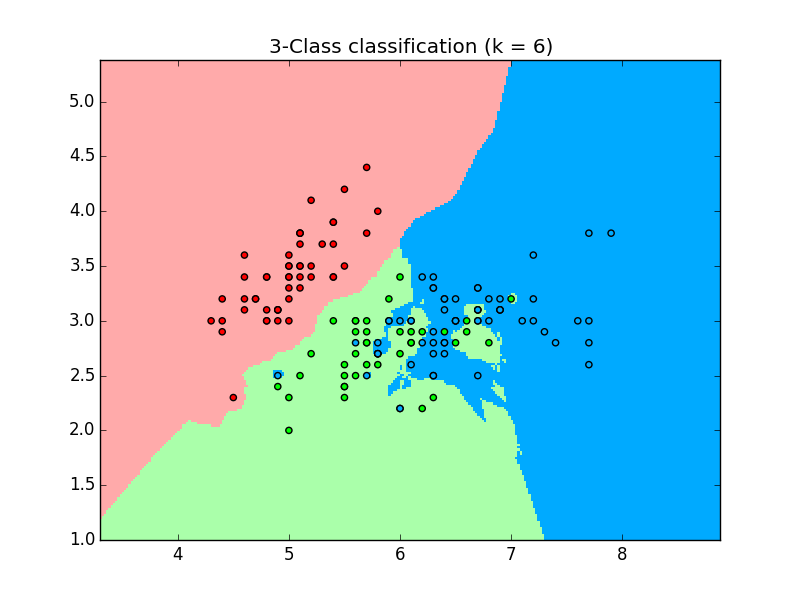# k nearest neighbors

Python hosting: Host, run, and code Python in the cloud!

Computers can automatically classify data using the k-nearest-neighbor algorithm.

For instance: given the sepal length and width, a computer program can determine if the flower is an Iris Setosa, Iris Versicolour or another type of flower.

Related courses

Dataset

Each plant has unique features: sepal length, sepal width, petal length and petal width. The measurements of different plans can be taken and saved into a spreadsheet.

The type of plant (species) is also saved, which is either of these classes:

• Iris Setosa (0)

• Iris Versicolour (1)

• Iris Virginica (2)

Put it all together, and we have a dataset:We load the data. This is a famous dataset, it’s included in the module. Otherwise you can load a dataset using python pandas.

X contains the first two features, being the rows sepal length and sepal width. The Y list contains the classes for the features.

Plot data
We will use the two features of X to create a plot. Where we use X[:,0] on one axis and X[:,1] on the other.

This will output the data:Classify with k-nearest-neighbor
We can classify the data using the kNN algorithm. We create and fit the data using:

And predict the class using

This gives us the following code:

which outputs the plot using the 3 classes:Prediction
We can use this data to make predictions. Given the position on the plot (which is determined by the features), it’s assigned a class. We can put a new data on the plot and predict which class it belongs to.

The code below will make prediction based on the input given by the user: